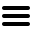vocabulary
1000+ books

# regression analysis used in Freakonomics

9 uses
(click/touch triangles for details)
Definition
statistics:  a method for determining the association between a dependent variable and one or more independent variables
• By subjecting it to the economist's favorite trick: regression analysis.
p. 163.3
• No, regression analysis is not some forgotten form of psychiatric treatment.
p. 163.3
• Regression analysis is the tool that enables an economist to sort out these huge piles of data.
p. 163.6
• In the case of the ECLS data, it might help to think of regression analysis as performing the following task: converting each of those twenty thousand schoolchildren into a sort of circuit board with an identical number of switches.
p. 164.1
• Regression analysis can't quite answer that question, but it can answer a subtly different one: does a child with a lot of books in his home tend to do better than a child with no books?
p. 164.7
• A regression analysis can demonstrate correlation, but it doesn't prove cause.
p. 164.9
• It should be said that regression analysis is more art than science.
p. 165.5
• Knowing what you now know about regression analysis, conventional wisdom, and the art of parenting, consider the following list of sixteen factors.
p. 168.5
• Using regression analysis to control for other factors that might influence life trajectories, it was then possible to measure the impact of a single factor—in this case, a woman's first name—on her educational, income, and health outcomes.
p. 191.4

There are no more uses of "regression analysis" in Freakonomics.

Typical Usage  (best examples)